# NCERT Solutions - Give And Take Notes | Study Mathematics for Class 3: NCERT - Class 3

## Class 3: NCERT Solutions - Give And Take Notes | Study Mathematics for Class 3: NCERT - Class 3

The document NCERT Solutions - Give And Take Notes | Study Mathematics for Class 3: NCERT - Class 3 is a part of the Class 3 Course Mathematics for Class 3: NCERT.
All you need of Class 3 at this link: Class 3

#### Page No 30:

Ques 1: Try these on Kittu’s home:
(a) 10 less than 34 is __________.
(b) 53 − 20 = _________
(c) 11 more than 31 is ________.
(d) 11 less than 66 is _________.
(e) 62 + 13 = ________
(f) 23 less than 89 is ________.
(g) 10 and 40 more is ________.
(h) 9 added to 28 gives ________.
(i) The sum of 9 and 44 is ________.
(j) Reducing 98 by 34 gives ________.
(k) 4 and 37 more is ________.
(l) Take 35 away from 83.
We get _______.
Ans: (a) We will start from 34. To find 10 less than 34, we will go 10 steps back or jump down one row. When we jump 1 row down, we reach 24. A jump from 34 to 24 is like taking 10 steps backwards from 34. We can write it like this: 34 − 10 = 24
Thus, 10 less than 34 is 24.
(b) We will start from 53. To subtract 20 from 53, we will jump 2 rows down. When we jump 2 rows down, we reach 33. A jump from 53 to 33 is like taking 20 steps backwards from 53. We can write it like this: 53 − 20 = 33
(c) We will start from 31. To find 11 more than 31, we will jump 1 row up from 31 and take 1 step right to reach 42. This is like taking 11 steps forward from 31. We can write it like this: 31 + 10 = 41 41 +1 = 42
Thus, 11 more than 31 is 42.
(d) We will start from 66. To find 11 less than 66, we will jump 1 row down from 66 and take 1 step left to reach 55. This is like taking 11 steps backwards from 66. We can write it like this: 66  − 10 = 56 56  − 1 = 55
Thus, 11 less than 66 is 55.
(e) We will start from 62. To add 13 to 62, we will jump 1 row up from 62 and take 3 steps right to reach 75. This is like taking 13 steps forward from 62. We can write it like this: 62 + 10 = 72 72 + 3 = 75
Thus, 62 + 13 = 75
(f) We will start from 89. To find 23 less than 66, we will jump 2 rows down from 89 and take 3 steps left to reach 66. This is like taking 23 steps backwards from 89. We can write it like this: 89 − 20 = 69 69 − 3 = 66
Thus, 23 less than 89 is 66.
(g) We will start from 10. To add 40 to 10, we can jump 4 rows up from 10. When we jump 4 rows up, we reach 50. A jump from 10 to 50 is like taking 40 steps forward from 10. We can write it like this: 10 + 40 = 50
Thus, 10 more than 40 is 50.
(h) We will start from 28. To add 9 to 28, we will jump 1 row up from 28 and take 1 step left to reach 37. This is like taking 9 steps forward from 28. We can write it like this: 28 + 10 = 38 38 − 1 = 37
Thus, 9 added to 28 gives 37.
(i) We will start from 44. To add 9 to 44, we will jump 1 row up from 44 and take 1 step left to reach 53. This is like taking 9 steps forward from 44. We can write it like this: 44 + 10 = 54 54 − 1 = 53
Thus, the sum of 9 and 44 is 53.
(j) We will start from 98. To reduce 98 by 34, we will jump 3 rows down from 98 and take 4 steps left to reach 64. This is like taking 34 steps backwards from 98. We can write it like this: 98 − 30 = 68 68 − 4 = 64
Thus, reducing 98 by 34 gives 64.
(k) We will start from 4. To add 37 to 4, we will jump 4 rows up from 4 and take 3 steps left to reach 41. This is like taking 37 steps forward from 4. We can write it like this: 4 + 40 = 44 44 − 3 = 41
Thus, 4 more than 37 is 41.
(l) We will start from 83. To take 35 from 83, we will jump 4 rows down and take 5 steps right to reach 48. This is like taking 35 steps backwards from 83. We can write it like this: 83 − 40 = 43 43 + 5 = 48
Thus, by taking 35 away from 83, we get 48.

#### Page No 34:

Ques 1: See if you can do the same with these sums.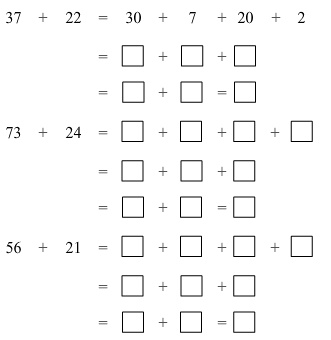Ans: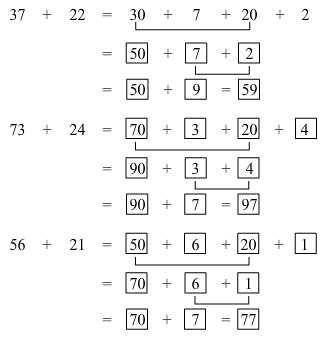#### Page No 35:

Ques 1: Now work out the steps in your mind. Write the answers directily in the boxes.
33 + 42 =
19 + 61 =
= 48 + 42
= 33 + 27
= 34 + 63
= 53 + 64
55 + 25 =
67 + 25 =
72 + 56 =

Ans:
33 + 42 = 75
19 + 61 = 80
90 = 48 + 42
60 = 33 + 27
97 = 34 + 63
117 = 53 + 64
55 + 25 = 80
67 + 25 = 92
72 + 56 = 128

#### Page No 40:

Ques 1: A shopkeeper Rafi had 153 candles. Paras gave him 237 more candles. How many candles does Rafi have now?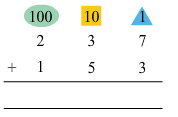Ans: Number of candles with Rafi = 153
Number of candles given by Paras to Rafi = 237
Total number of candles with Rafi = 237 + 153 = 390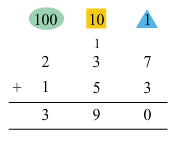Thus, Rafi has a total of 390 candles.

Ques 2: A train compartment is carrying 132 people. Another compartment is carrying 129 people. In all, how many people are there in both the compartments?
Ans: Number of people in the first compartment = 132
Number of people in the second compartment = 129
Total number of people in both the compartments = 132 + 129 = 261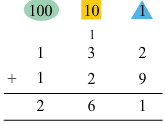Thus, there are 261 people in both the compartments.

#### Page No 41:

Ques 1: Shanu found 138 pebbles. Karim found 44 pebbles. How many pebbles did they find in all?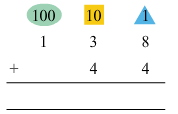Ans:
Number of pebbles with Shanu = 138
Number of pebbles with Karim = 44
Total number of pebbles = 138 + 44 = 182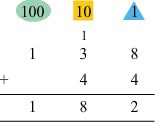Thus, Shanu and Karim found 182 pebbles altogether.

Ques 2: A teacher kept a note of which fruits students like in her school. This is what she found: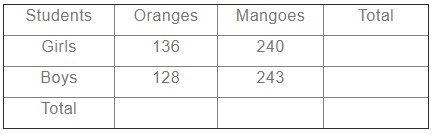Find out:
(a) How many students in the school like oranges?
(b) How many students in the school like mangoes?
(c) Altogether, how many students are there in the school?
(d) Is the number of girls more than 350 or less than 350?

Ans: (a) Number of girls who like oranges = 136
Number of boys who like oranges = 128
Total number of students who like oranges = 136 + 128 = 264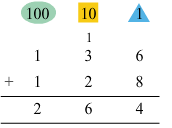(b) Number of girls who like mangoes = 240
Number of boys who like mangoes = 243
Total number of students who like mangoes = 240 + 243 = 483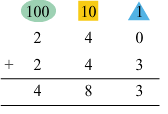(c) Total number of students in the school = 264 + 483 = 747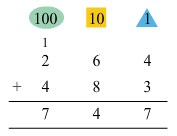(d) Number of girls who like oranges = 136 Number of girls who like mangoes = 240 Total number of girls in the school = 136 + 240 = 376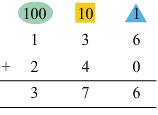Thus, the number of girls in the school is more than 350.

#### Page No 42:

Ques 1:
A. (i) 345 + 52 (ii) 492 + 29 (iii) 245 + 93 (iv) 643 + 345 (v) 750 + 219
B.
319+ 823
804+ 406
363+ 456
427+ 248
684+ 232

Ans: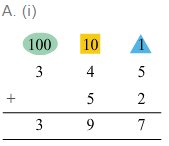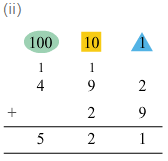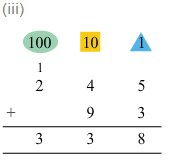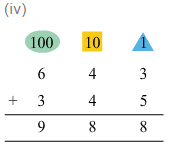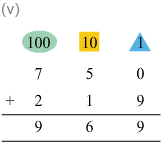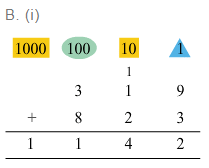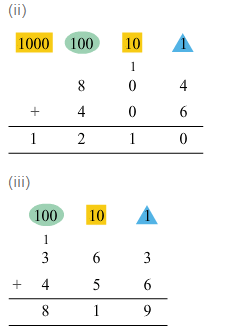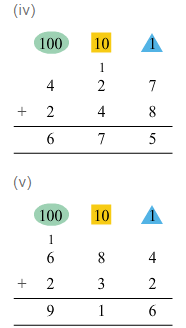#### Page No 44:

Ques 1: Do all the sums mentally:
(a) 75 + 20 = 95
(b) 90 + 60 = 150
(c) 25 + 30 + 3 =
(d) 9 + 40 + 31 =
(e) 500 + 200 =
(f) 400 + 350 =
(g) 670 + 120 =
(h) 380 + 210 =
(i) 205 + 650 =
(j) 128 + 600 =
(k) 150 + 69 =
(l) 37 + 46 + 3 =

Ans: (a) 75 + 20 = 95
(b) 90 + 60 = 150
(c) 25 + 30 + 3 = 58
(d) 9 + 40 + 31 = 80
(e) 500 + 200 = 700
(f) 400 + 350 = 750
(g) 670 + 120 = 790
(h) 380 + 210 = 590
(i) 205 + 650 = 855
(j) 128 + 600 = 728
(k) 150 + 69 = 219
(l) 37 + 46 + 3 = 86

#### Page No 45:

Ques 1: You can also play it. Here are the cards for you. Work out the combination. Place the cards in the right boxes.
(a)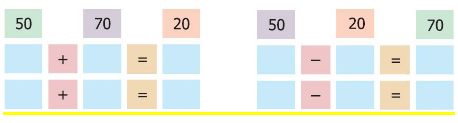(b)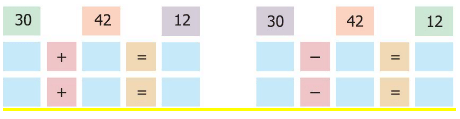Ans:
(a)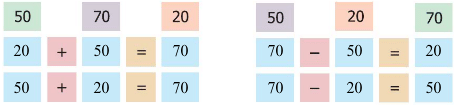(b)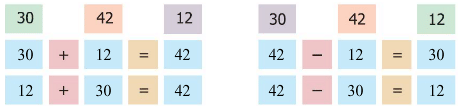The document NCERT Solutions - Give And Take Notes | Study Mathematics for Class 3: NCERT - Class 3 is a part of the Class 3 Course Mathematics for Class 3: NCERT.
All you need of Class 3 at this link: Class 3Use Code STAYHOME200 and get INR 200 additional OFF

## Mathematics for Class 3: NCERT

57 videos|70 docs|44 tests

Track your progress, build streaks, highlight & save important lessons and more!

,

,

,

,

,

,

,

,

,

,

,

,

,

,

,

,

,

,

,

,

,

;